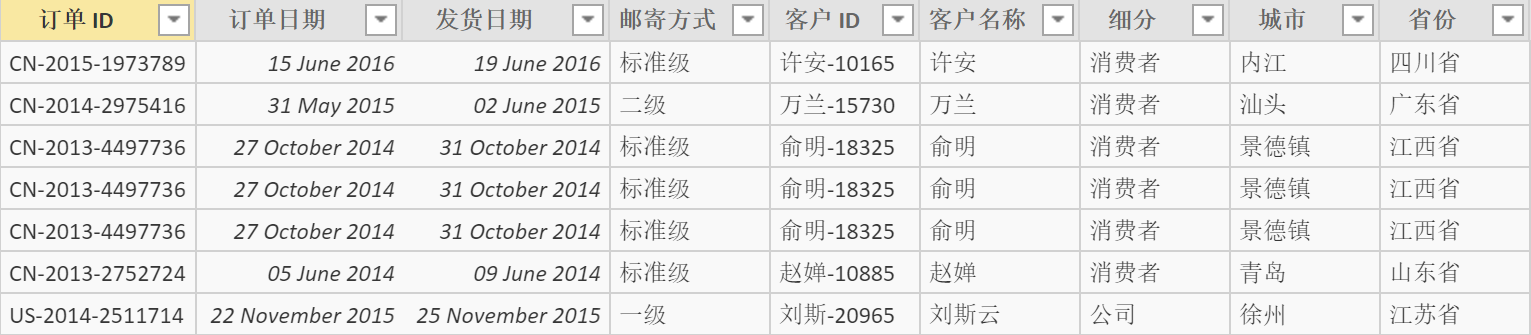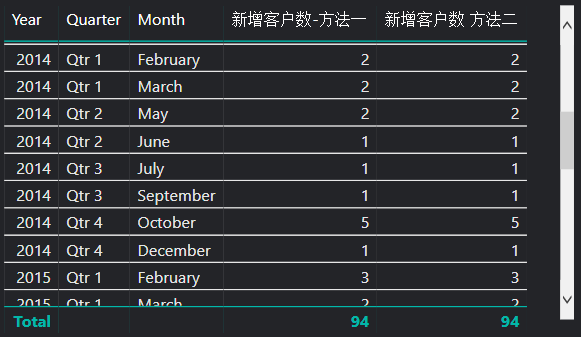# 两种方法计算每月开发的新客户数量

## 过程``````二次购买判断 =
VAR
E_Date = 'Data'[订单日期]
VAR
CUST = 'Data'[客户 ID]
RETURN
IF(
SUMX(
FILTER('Data',CUST = 'Data'[客户 ID]&&E_Date > 'Data'[订单日期]),
COUNTROWS('Data'))>0,"非首次","首次")
``````

``````每月新增客户数 =
CALCULATE(
DISTINCTCOUNT(Data[客户 ID]),'Data'[二次购买判断] = "首次")
``````

``````每月新增客户数 2 =
VAR
X = -1
VAR
CUST = VALUES('Data'[客户 ID])
VAR
Pre_CUST =
CALCULATETABLE(VALUES('Data'[客户 ID]),
FILTER(ALL('Data'),
&&'Data'[订单日期]<MIN('Data'[订单日期])))
RETURN
COUNTROWS(
EXCEPT(CUST,Pre_CUST))
``````## 其他